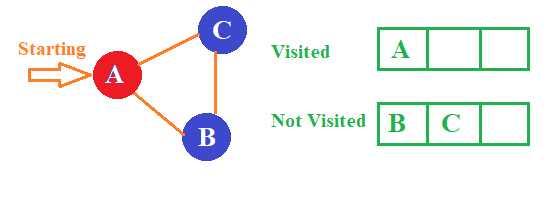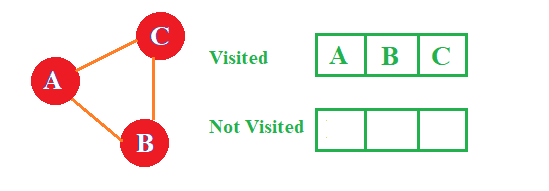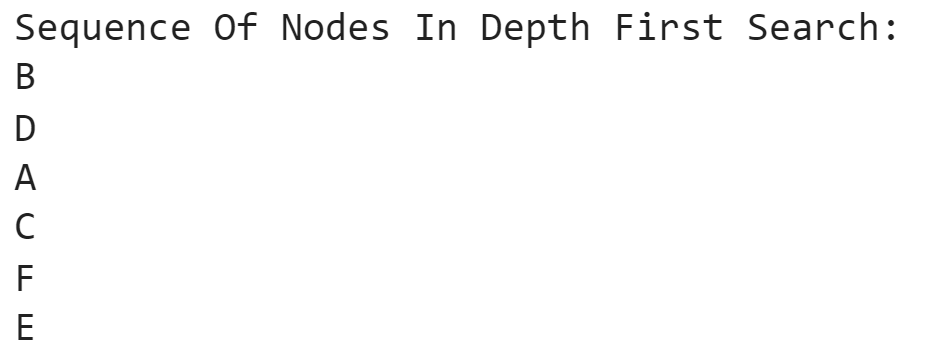# How To Implement Depth First Search In Python ? Code Explained

But before we start writing about DFS Python, let us try to understand the necessity of DFS in Python by analyzing one daily life example. This example will help to understand the importance & the concept of DFS Python more clearly.

##### Real-life Example of Depth First Search In Python:

Suppose, you have visited a fair near to your house. At that fair, there are many attractive rides are present. in that fair, you have identified one magical room. In that magical room, there are lots of ways out are present to exit from that maze.  You find it an interesting one. So, you try to find out the possible way to move out of the maze. But you tried a lot to find it out. But you can’t able to do that.

So, what will you do? Do you have any idea to move out of the maze?

So, you thought about the possible way to come out & found an idea. You start marking on each & every way. If the way is leading to the exit point it will be better. Otherwise, you will mark them as negative ones. In this way, you can able to reduce the number of ways present in that maze. And eventually, you will come out of the maze. The same thing goes for the DFS in Python.

In DFS Python, there will be different nodes present. there is a need to start a journey from any of the nodes & need to traverse all the nodes present in the tree or graph. In Depth First Search Python, there is a need to mark all the nodes as visited or not. We will learn more about it when we start discussing the implementation of DFS in Python. But let us try to understand more about the Depth First Search Python.

Also, if you’re considering to learn more about Python, then you can read our article ‘ Stack vs Python: Which Is Better For Python?’

## What Is Depth First Search Python? Get To Know

DFS Python is a  concept that relates to the Data Structure concept. DFS in Python is developed on the Data Structure concept graphs & trees. In DFS Python, there is a need to take the graph or tree for more operations. In the DFS Python, there is a need to mark all the nodes present in the graph as visited or not. There is a need to visit all the paths present in the DFS Python. The DFS in Python works on the recursive method. That means, one function will call itself multiple times till the ending condition is satisfied. DFS in Python also uses a special operation. This operation is known as Backtracking.

Backtracking is a special method in the Recursion process. The name itself suggests that something backward is happening in this process. The main goal of this process is to move towards a special direction until there is a dead end.

The backtracking process helps to move ahead in any special direction. If there is no way to move ahead, then it will use the same path to come back to the originated node. DFS in Python uses this special operation for implementing the programming. The concept of DFS Python will be clear when we discuss its theory of it.

We all are aware how important learning Python is, but still if you want to know more then you can check out our article on “5 Reasons Why To Learn Python“.

## What Is The Theory Of Depth First Search Python? Get To Know

Before we start discussing the DFS in Python, there is a need to understand the theory of DFS Python. Before going to discuss the theory, there is a need to clarify that some Data Structure concept is going to be used here. You need to have a very strong hold on the Data Structure concept. In this theory, there is a need to use a Graph concept & the Stack concept.

The graph will help to imagine the placement of the nodes. It will help to identify which node is adjacent to which node. Along with the graph, there is a need to use the Stack & Array. In Array, there is a need to store the nodes that are visited. And in Stack, there is a need to store the nodes that are not visited yet. From this, we are going to find some similarities with our daily life experiences.

• ##### Step 1: Starting The Traversing

Suppose, we have the following graph where three nodes are present. All three nodes are adjacent to each other. Now, there is a need to implement one Depth First Search Python to this graph. For that purpose, there is a need to start traversing. Here, we are traversing the graph from the A node. And remaining two nodes are not visited till now, so they are placed in the Stack one by one. And A node will be saved to the array as it is visited.• ##### Step 2: Going To Unvisited Nodes

Now, in the stack, all the nodes are adjacent to the starting node. The uppermost node present in the Stack will be popped out first. And it will be visited. As this node is now visited it will be marked & save to the array. So, in the stack, only one node is present that is unvisited.• ##### Step 3: Ending The Process With The Last Remaining Node

So, the remaining node that is present in the stack will be now visited. As this node is now adjacent to the current node. So, this remaining node will be removed from the stack & will be placed in the array by marking it as Visited. Hence, we have completed traversing in the Depth First Search Python. And the total sequence of the nodes is now available with output.### What Is The Algorithm Of Depth First Search Python?

After clearly understanding the theory of Depth First Search Python, there is a need to understand the algorithm of Depth First Search Python. Depending upon the algorithm, there is a need to implement the process to find out Depth First Search Python. The algorithm is the process where we try to understand the implementation process of any code. It acts as the blueprint of any programming.

Let us try to understand the algorithm of Depth First Search Python:

Step 1: At first, there is a need to provide the nide index number to the program to indicate the starting point. From that point, all the traversing will be done.

Step 2: After that, there is a need to place all the adjacent nodes to the stack & mark them as unvisited. Also, the node that is currently visited needs to be placed in the array.

Step 3: Then there is a need to remove the uppermost element from the stack & mark it as the visited one. Then we will need to focus on the second element in the stack.

Step 4: There is a need to execute Step 2 & Step 3 until the stack goes empty & there is no unvisited nodes are present in the graph.

### What Are The Applications Of Depth First Search Python?

After getting the algorithm of Depth First Search Python, there is a need to move to the implementation process of Depth First Search Python. But there is a need to first understand its necessity in real life. There is a need to understand the applications of Depth First Search Python in our everyday life. For that, there is a need to focus on the following list.

• The Depth First Search Python is mostly used to find out any cycles present in the graph or not. The presence of any cycle in any graph is not an acceptable one.
• The Depth First Search Python is mostly used in the maps to find out the paths. Using that, we can easily move to the destination without having any issues.
• The Depth First Search Python helps to find out the nodes that are strongly connected in any graph. Depending upon that, many more concepts can be developed.
• The Depth First Search Python is used to solve any problem that should have only one solution among all the possible solutions. It helps to solve puzzles also.
• The Depth First Search Python also helps to analyze the network concept. The networks also are connected using some nodes. It helps in that case also.

Looking to gain some more knowledge in the programming world and are curious to know more about binding constraints then you can check out our article

### What Is The Implementation Process Of Depth First Search Python?

Moreover, we have completed all the necessary pieces of information present in Depth First Search Python. Now, we have come to the end of our journey. Here, we are going to implement the program for Depth First Search Python. It is the most important part of this article. Readers are advised to pay full attention here to completely understand the process.

At first, there is a need to declare a list of nodes. Inside that big list, there is a need to declare some other list also. That list will indicate the adjacent nodes present with each other. The outside one will be the main one. And inside the node, there will be a list of adjacent nodes. After that, there is a need to declare one set that will track all the records of the visited nodes in Depth First Search Python. After that, there is a need to declare one function to do all operations.

Inside that function, there is a need to define a condition that will check which nodes are unvisited. Then the very adjacent node will be printed & it will be added as the visited one. Then there is a need to call the function in recursive format to find all the nodes’ sequences & print them. When there all the nodes will be checked with the function will be terminated.

Along with that, there is a need to implement the main part of the programming. There we are going to first write down some comments before printing the sequence of the nodes.  After that, there is a need to call the function to start the total process. While calling the function, there is a need to share the starting node name along with the function. Then we are all set to get the output.

Now, there is a need to look at the full code of the Depth First Search Python. There is a need to understand the code properly. It will help to understand the necessity of Data Structure in programming. There is a need to know the basics of programming to understand this concept more clearly & easily.

##### Example:

```nodes ={'A' : ['B','C'],'B' : ['D', 'E'],'C' : ['F'],'D' : ['A'],'E' : ['F'],'F' : []} # List Of Nodes With Adjacent Nodes

checked = set() # Declaration Of Set Of Elements To Get Record Of Visited Nodes

def dfs(checked, nodes, n): # Function For Getting The Sequence Of Nodes & Printing The Results

if n not in checked: # Condition For Checking The Not Visited Nodes In The List

print (n) # Printing The Nodes For Developing The Sequence

for adjacent in nodes[n]: # For Loop To Check Different Other Adjacent Nodes

dfs(checked, nodes, adjacent) # Recursively Calling The Function To Complete The Process

# Main Code

print("Sequence Of Nodes In Depth First Search:") # Printing The Statement Before Sequence

dfs(checked, nodes, 'B') # Calling The Function & Starting The Program```

Let us try to find out the output of the above code. It will help to understand the implementation process of Depth First Search Python.

##### Output:## Conclusion:

As we saw, the Depth First Search Python is a very important concept. There is a necessity to know about this concept before moving to any advanced topic in Python programming language.

We need to remember the algorithm to develop the Depth First Search in Python programming language. Using the algorithm, you can easily develop the programming in Python programming language. Also, we need to remember the applications of the Depth First Search in Python programming language.

There is a need to clear the basic concept of Python programming language before going through this topic. Along with that, it is advisable to clear the concept of the Data Structure before starting to implement this process. We need to understand the importance of this topic. Practicing this topic more & more will help us in the future.

So, hope you have liked this piece of article. Share your thoughts in the comments section and let us know if we can improve more.